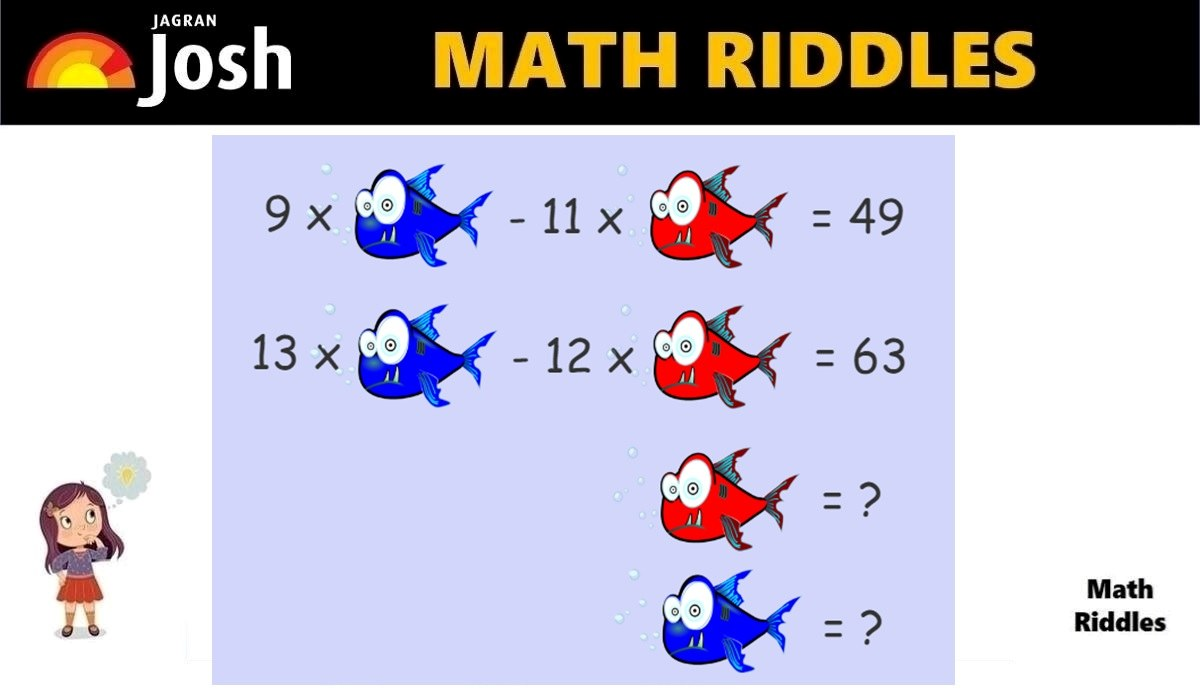# Math Riddles With Answers: Can You Find The Value of Red Fish and Blue Fish in 20 Seconds?

In this Math Puzzle, we have to Can you find the values of Red Fish and Blue Fish each to solve this hard math riddle. Are you a math genius? Only 1% with a high IQ can solve this tricky Fish Logic Math Puzzle in 20 seconds.Math Riddles With Answers: Can You Find The Value of Red Fish and Blue Fish in 20 Seconds?

In this Math Puzzle, we must find the values of Red Fish and Blue Fish each to solve this tricky math riddle. Are you a math genius? Only 1% with a high IQ can solve this tricky math puzzle in 20 seconds.

Share this math puzzle with your friends, kids, students, colleagues, and family. These math riddles are a great source for solving math problems in a fun way. Try to test your mathematical knowledge and genius level with this math puzzle. Let us solve this math picture puzzle within 20 seconds.

If you love solving cryptic math puzzles, give this math puzzle a try! You have 20 seconds to find the correct answer.

## Math Riddles with Answers: Solution

Answer: Blue Fish = 3 and Red Fish = -2

Explanation:

We shall refer to the Blue Fish as X and Red Fish as Y.

Let us look at the equations in a mathematical way.

9X – 11Y = 49 ………………. (Equation 1)

13X – 12Y = 63 ……………….. (Equation 2)

We will solve the question using the elimination method.

We will have to make the X- coefficients the same by eliminating the terms with 'X'.

Picture Puzzles: Only 1% Genius Can Find The Hidden Words In This Living Room Within 11 Seconds

We will multiply equation (1) with 13 and equation (2) with 9, and then we will subtract equation (2) from (1).

=> 13 x (9X – 11Y) = (49) x 13 ………. Equation 1

=> 9 x (13X – 12Y) = (63) x 9 ……….. Equation 2

We get,

=> 117X – 143Y = 637

=> 117X – 108Y = 567

Let us subtract these both equations. (We shall subtract the LHS of Equation 1 from the LHS of Equation 1 and RHS of Equation 2 from the RHS of Equation 2).

=> 117X – 143Y - (117X – 108Y) = 637 -567

=> 117X – 143Y – 117X + 108Y = 70

Both 117X and -117X cancel each other.

Picture Puzzles Only 1% Visually Intelligent Can Spot The Toilet Paper Roll Within 11 Seconds

We now get:

=> -143y +108y = 70

=> -35y = 70

=> y = -2

Now, let us put the value of Y in equation (1) and solve to find the value of X

=> 9X – 11Y = 69

=> 9X- 11(-2) = 49

=> 9X +22 = 49

=> 9X = 49 -22

=> 9X = 27

=> X = 3

Therefore, the values of X (which is Blue Fish) is 3 and Y (which is Red Fish) -2 respectively.

Great Work!

## Tell us in comments: Did you solve this math puzzle correctly in 20 seconds?

Check out more amazing tricky math puzzles!

Math Riddles With Answers: Solve This Donald Duck, Jerry, Mickey Mouse Math Puzzle in 20 Seconds

Can You Solve This Tricky Flower Math Riddle In 20 Seconds?

Math Riddles With Answers: Can You Find The Value Of Lemon In 20 Seconds?

Personality Test: Your sleeping position reveals these personality traits